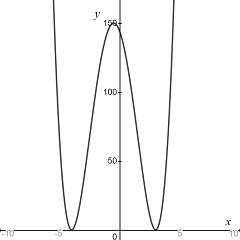# Sketch the graph for a single function that has all of the properties listed. f'(-4)=f'(3)=0

## Question:

Sketch the graph for a single function that has all of the properties listed.

{eq}f'(-4)=f'(3)=0 {/eq}

## Relative Extrema:

A function of one real variable {eq}f(x) {/eq} has a critical point at {eq}x=a {/eq}

if the following condition holds:

{eq}f'(x)=0. {/eq}

The critical point is a relative minimum for f if the second derivative at that point is positive.

The critical point is a relative maximum for f if the second derivative at that point is negative.

The critical point is a neither a maximum or a minimum is the second derivative at that point is zero.

We know that a function f(x) has two critical points at {eq}x=-4, \; x=3 {/eq} that is

{eq}f'(-4)=f'(3)=0 {/eq}

We assume that the critical points are relative minima. Then, a function with these properties is

{eq}y = (x+4)^2(x-3)^2 {/eq}

whose graph is illustrated in the figure below.